## Welcome to the World of Modelling and Simulation

### What is Modelling?

This blog is all about system dynamics modelling and simulation applied in the engineering field, especially mechanical, electrical, and ...### Bond Graph Modelling, A Quick Learning: Part 3

In this part, I am going to present step by step methods to build a bond graph model from scratch for the following mass-spring-damper model which was shown in the previous part-2. However, before that, I need to discuss the nine fundamental elements of the bond graph first. This material is adapted from my dissertation and following two references [1, 2]; if you are interested to dig deeper in this field, I would strongly suggest to go through the references.

Fundamental Elements:
The fundamental elements of bond graph are source of effort (SE), source of flow (SF), inductive element (I), capacitive element (C), resistive element (R), transformer (TR), gyrator (GY), 1-junction, and 0-junction. Here, I am including a table which reflect the analogy of bond graph element in different energy domains.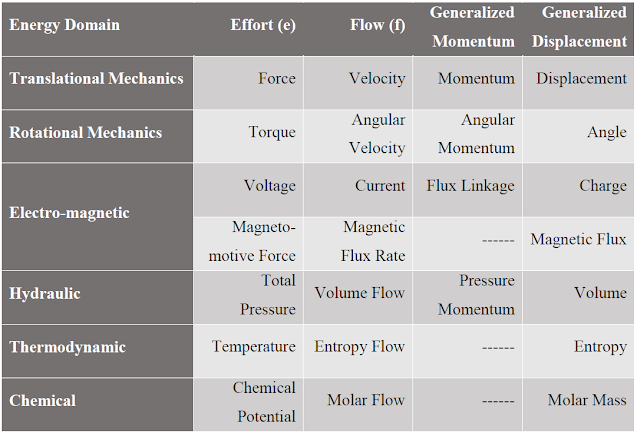Table 1: Analogies of the Bond Graph Variables Utilized in Different Domains 

In bond graph, each element is connected by bonds, and energy is transferred through the bond. The energy or power is composed of two things: effort (e) and flow (f). The multiplication of these two provides the power. Fig. 1 illustrates the exchange of information between two elements.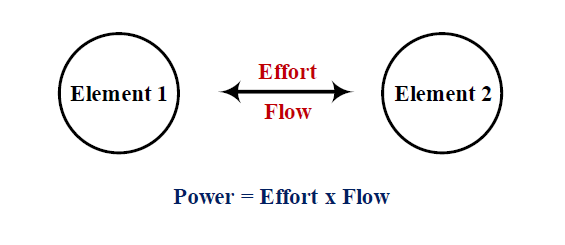Fig. 1: Elements share information through bond
Direction of the energy exchange through the power bonds between any of the two elements are determined by causality. So, the term causality informs us which side of a power bond between two elements determines the instantaneous effort and which determines the instantaneous flow. A causal stroke is a small perpendicular line either attached at one end of the bond or port line. Causal stroke exemplifies the direction of power flow for each of the elements acting on the system.

Fig. 2 shows a causal stroke which is a small perpendicular line attached at one end of the bond. The half arrow between two elements in Fig. 2 signifies the bond. The causal stroke creates the direction of energy flow from element 1 to element 2.Fig. 2: Power direction and causality between elements

Inductive Element:
The inductive or I-elements are energy storage elements. They store all kinds of free energy. The variable (p-type) of an inductive element is a conserved quantity which is the result of an integration operation and is also a state variable of the system. In this case, the conserved quantity p is stored by accumulating the net effort to the element. The natural causality of an inductive element is to take effort for flow generation. Examples of inductive elements are mass in mechanical domain, inductor in electrical domain and so on. In bond graph representation, the natural causality of an inductive element with its symbol and causal stroke is represented by:

Capacitive Element:
Similar to inductive elements, capacitive or C-elements are energy storing elements. In C-element, the conserved quantity (q-type) is stored through accumulating the net flow. So, its natural causality is to take the flow information to generate the effort information which is unlike the inductive element. For instance, the capacitive element is a capacitor in electrical domain, a spring in mechanical domain, a water tank in hydraulic domain etc. In bond graph, the representation of a capacitive element is,

Resistive Element:
Unlike inductive or capacitive elements, resistors or R elements are the energy dissipating elements. And, the constitutive equation for this element is an algebraic relation between effort and flow. Resistive elements are for example dampers in mechanical domain, electric resistors in electrical domain and so forth. In bond graph representation, the natural causality of a resistive element with its symbol and causal stroke is represented by:

Source of Effort and Flow:
An effort or a flow source signifies its interaction with the environment. An effort element connected to a system provides the energy to that system. An effort element is an externally determined quantity which if connected through bonds does not rely on the rest of the elements of a system. For example, effort sources are torque, force in mechanical domain; voltage, magneto motive force in electro-mechanical domain and so on. On the other hand, it depends on the rest of the system to decide the flow. So, flow through any bond is not determined by the external elements while this is up to the system to decide the flow. As for example, flow source in mechanical domain is velocity; in electrical domain is current and so forth. The flow and effort sources are represented by symbols SE and SF.

Junction-1 and 0:
and 0 junctions adjoin two or more elements in a power continuous way (no absorption or dissipation of power in junctions). At 1-junction, flows are distributed equally and at 0-junction, efforts are distributed equally. For this reason, these two junctions are also called flow equalizing and effort equalizing junctions accordingly. In bond graph representation, the natural causality of a and 0 junctions with its symbol and causal stroke are represented by:

Transformer:
Transformer or TR element determines the conversion of energy in the system. Transformer transfigures flow into flow or effort into effort in a system. Besides, it is a power continuous element (no absorption or dissipation of power). The following notation shows how a transformer is represented in a bond graph model with its causality.

Gyrator:
Gyrator or GY element also converts energy in bond graph analogous to transformer. But, this element converts flow into effort or effort into flow. Similar to transformer, there is no energy loss during the conversion. An electric motor or a generator may be the best example to illustrate the gyrator. The bond graph representation of a gyrator is,

Development of a Bond Graph Model for the Mass-Spring-Damper System:
Now, we are ready to discuss the step-by-step procedure to construct a bond graph model for the system shown in Fig. 3. To begin, the junctions of the system are identified where the velocity or force of the connected elements remain same. The red dots abc, and d in Fig. 4 represent those points or junctions.Fig. 3: Physical mass-spring-damper system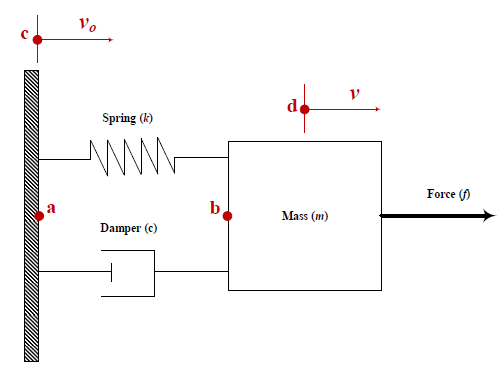Fig. 4: Junctions identification

Points c and d can be represented by 1-junctions where two distinct velocities (v and vo) are identified in Fig. 5. Here, v is the velocity of the mass and vo is the velocity of the wall which is zero in this case since the wall is not moving, However, for the initial construction of the bond graph, it would be helpful to consider this reference zero velocity that can be eliminated at later time.Fig. 5: 1-junctions defining the velocities
Next step is to identify the elements that share the same flow connected to those 1-junctions. From Fig. 4, we see that the mass and effort source (force (f)) share the same flow. So, we may connect those two elements to a 1-junction where the velocity is labeled as v with power direction as well as causality. And, to the other junction with designated velocity vo, a flow source element may be attached which would represent the wall as the flow is considered zero. Following Fig. 6 illustrates the development where mass is represented by I, force is represented by SE and SF represents the wall having no velocity.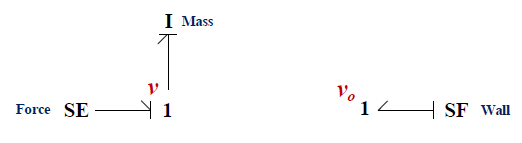Fig. 6: Elements attached to 1-junctions that have the same velocity
In Fig. 4, two more points were identified. Those are points a and b where the effort remains same. We may link the two segments shown in above Fig. 6 with a 0-junction since they share the same effort. Moreover, spring and damper have the same flow, so these two elements may be connected through another 1-junction in the bond graph. Fig. 7 highlights this aspect where the 0-junction between two segments from previous figure is considered as the effort is same through points a to b in Fig. 4 while 1-junction signifies that the spring and damper elements share the same velocity. Now, the final step is to connect rest of the system through these junctions with power bond maintaining the causality between elements as before. It is a good practice to mark each bond in the model (Fig. 8) with distinct number so that we can instantly identify or name the element by bond number that is connected to the respective bond. Moreover, the strategy will also help to extract differential equations from bond graph model very conveniently which is shown in the following section.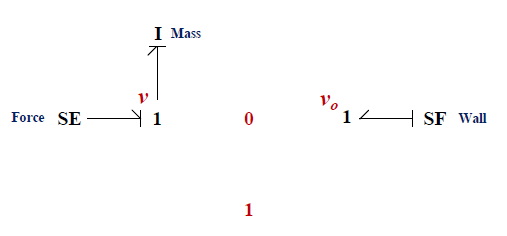Fig. 7: Two more junctions implemented to link rest of the elements
Fig. 8 shows the complete bond graph model of the overall system depicted in Fig. 3. The bond graph model is such a graphical representation which is more intuitive to understand the dynamics of the system essentially based on the constitutive relations of the elements. The source of effort (SE) herein is the applied force (f) on the mass that is obviously the energy entry into the system. This energy is then exchanged at once by the inductive, capacitive, and damping elements based on the causality. Now, if you can build this diagram, you no longer need to derive the system differential equation. There are many dedicated bond graph software which can handle the generation of the equations based on the bond graph information.
Although, it is not necessary to extract the differential equations from the following bond graph model; however, for the beginners, it would be very interesting to see that we can eventually come up with the same differential equation that we use to derive by sketching a free body diagram. That part is left for now, and will be discussed in the next session. Until then, try to develop very simple bond graph model using the technique described in this article.Fig. 8: Complete bond graph model of the mass-spring-damper system

REFERENCES

 Karnopp, D. C., Margolis, D. L., and Rosenberg, R. C., 2012, “System Dynamics: Modeling and Simulation of Mechatronic Systems”, Fifth Edition, John Wiley and Sons, Inc.

 Borutzky, W., 2010, “Bond Graph Methodology: Development and Analysis of Multi Disciplinary Dynamic System Models,” Springer-Verlag London Limited.